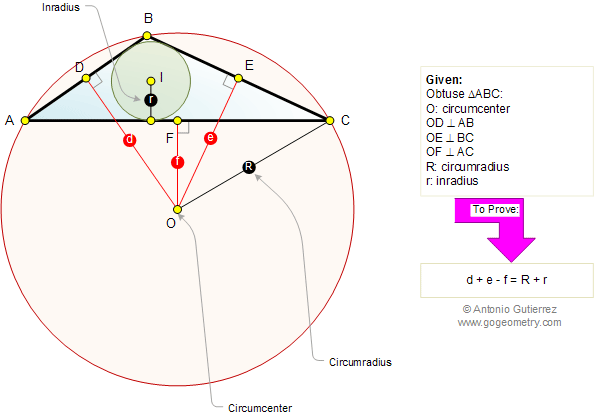# Online Geometry: Carnot's Theorem in an Obtuse Triangle

In an obtuse triangle ABC (angle B is obtuse), d, e, and f are the distances from the circumcenter O to the sides AB, BC, and AC, respectively. If R is the circumradius and r the inradius, then d + e - f = R + r.Home | Geometry | Search | Problems | Classical Theorems | Perpendicular Bisector | Email | Post a comment or solution | By Antonio Gutierrez